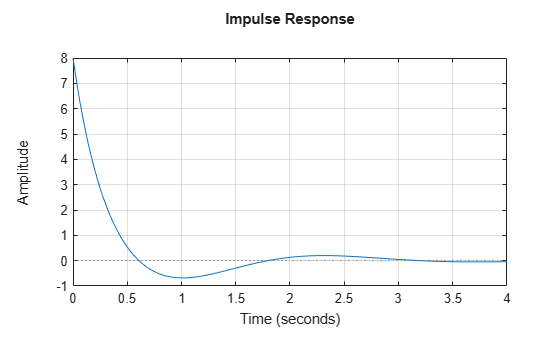Documentation

## Time-Domain Response Data and Plots

This example shows how to obtain step and impulse response data, as well as step and impulse response plots, from a dynamic system model.

Create a transfer function model and plot its response to a step input at $t$ = 0.

```H = tf([8 18 32],[1 6 14 24]); step(H);```When call `step` without output arguments, it plots the step response on the screen. Unless you specify a time range to plot, `step` automatically chooses a time range that illustrates the system dynamics.

Calculate the step response data from $t$ = 0 (application of the step input) to $t$ = 8 s.

`[y,t] = step(H,8);`

When you call `step` with output arguments, the command returns the step response data `y`. The vector `t` contains corresponding time values.

Plot the response of `H` to an impulse input applied at $t$ = 0. Plot the response with a grid.

```opts = timeoptions; opts.Grid = 'on'; impulseplot(H,opts)```Use the `timeoptions` command to define options sets for customizing time-domain plots with commands like `impulseplot` and `stepplot`.

Calculate 200 points of impulse response data from $t$ = 1 (one second after application of the impulse input) to $t$ = 3s.

`[y,t] = impulse(H,linspace(1,3,200));`

As for `step`, you can omit the time vector to allow `impulse` to automatically select a time range.